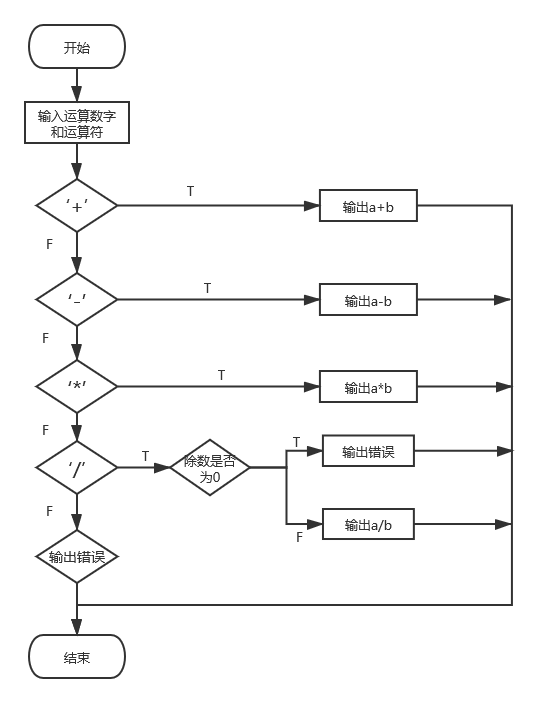# c语言程序设计实验报告（三）

2020-03-26 13:05:34  阅读：17  来源： 互联网

# 一、实验目的与要求

1、掌握c语言逻辑值的表示方法（0代表“假”，1代表“真”）
2、学会正确的使用关系表达式和逻辑表达式
3、掌握各种形式的if语句语法和使用方法。if语句中if和else的匹配关系，以及if语句的嵌套
4、熟练掌握switch语句的语法和使用方法，注意switch语句中break语句的用法及switch语句的嵌套

# 二、实验内容

## 1、实验练习： 实验4.3.1 if语句的应用

1问题的简单描述：读入3个表示箱子的长、宽、高的整数值，判断并输出该箱子是正方体还是长方体。
2实验代码：

``````#include<stdio.h>
main()
{
int l,w,h;
printf("请输出箱子的长、宽、高;\n");
scanf("%d%d%d",&l,&w,&h);
if((l==w)&&(w==h))
printf("该箱子为正方形。\n");
else
printf("该箱子为长方形。\n");
return 0;
}
``````

3问题分析：最初编写代码时使用的时l=w=h，发现不可以，后改用了(lw)&&(wh)的逻辑表达式

## 2、实验练习： 实验4.3.2 switch.....case语句的应用

1问题的简单描述：编写程序实现以下功能：商店有打印纸（18元/本）、墨盒（132元/个）、光盘（4.5元/张）出售，商店进行优惠酬宾 活动。具体规则如下：顾客购买商品的总额超过100元，优惠5%；超过200元，优惠6%；超过300元，优惠7%；超过400元优惠8%；超过500元，优惠10%。根据不同的购买量，计算应付贷款。
2实验代码：

``````#include<stdio.h>
main()
{
int x,y,z,a;   //x为打印纸的数量，y为墨盒的数量，z为光盘的数量
float sum;
sum=0.0;
printf("请输入打印纸、墨盒、光盘的数量:\n");
scanf("%d%d%d",&x,&y,&z);
sum=18*x+132*y+4.5*z;
a=sum/100;
switch(a)
{
case 1:
sum=0.95*sum;break;
case 2:
sum=0.96*sum;break;
case 3:
sum=0.93*sum;break;
case 4:
sum=0.92*sum;break;
default:
sum=0.9*sum;break;
}
printf("应付款=%.2f\n",sum);
return 0;
}
``````

3问题分析：刚开始时对于如何对于不同折扣的判断有些不知道怎么办。

## 3、实验练习： 实验4.3.3 switch....case语句嵌套if语句的应用

1、问题的简单描述：输入某一年、月的数值，输出该月的天数。
2、实验代码：

``````#include<stdio.h>
int main()
{
int year,month,days;
printf("plaese enter year and month:\n");
scanf("%d%d",&year,&month);
switch (month)
{
case 2:
if(((year%4==0)&&(year%100!=0))||(year%400==0))
days=29;
else
days=28;
break;
case 1:
case 3:
case 5:
case 7:
case 8:
case 10:
case 12: days=31;break;
case 4:
case 6:
case 9:
case 11: days=30;break;
}
printf("%d年%d月有%d天",year,month,days);
return 0;
}
``````

3、问题分析：无

## 4、实验练习： 实验4.3.4 switch....case结构嵌套的应用

1、问题的简单描述：编写一个自动售货机的程序。该程序具有如下功能：有两个二级菜单，一级菜单是商品类型的选择；二级菜单是具体商品的选择。顾客先选择商品的类型，然后选择商品，输入购买数量.自动售货机根据选择的商品和输入的数量，计算并显示所选商品的总金额。
2、实验代码：

``````#include<stdio.h>
int main()
{
int x,n,y;
float sum=0.0;
printf("请选择：1.日用品  2.文具  3.食品\n");
scanf("%d",&x);
switch (x)
{
case 1:printf("请选择：1.牙刷（3.5元/支） 2.牙膏（6.2元/支）\n");
printf("        3.肥皂(2元/块)   4.毛巾（8.6元/条）\n");
scanf("%d",&y);
printf("数量？");
scanf("%d",&n);
switch (y)
{
case 1:sum=3.5*n;break;
case 2:sum=6.2*n;break;
case 3:sum=2*n;break;
case 4:sum=8.6*n;break;
}
break;
case 2:printf("请输入:1.笔（3元/支）  2.笔记本（1.2元/个）\n");
printf("       3.文件夹（12元/个）  4.文具盒（8.6元/个）\n");
scanf("%d",&y);
printf("数量?");
scanf("%d",&n);
switch (y)
{
case 1:sum=3*n;break;
case 2:sum=1.2*n;break;
case 3:sum=12*n;break;
case 4:sum=8.6*n;break;
}
break;
case 3:printf("请输入：1.白糖（3.6元/包）  2.盐（1元/包）\n");
printf("        3.饼（2元/个）   4.方便面（3.6元/包\n");
scanf("%d",&y);
printf("数量？");
scanf("%d",&n);
switch (y)
{
case 1:sum=3.6*n;break;
case 2:sum=1*n;break;
case 3:sum=2*n;break;
case 4:sum=3.6*n;break;
}
break;
}
printf("总计:%.2f元\n",sum);
return 0;
}
``````

3、问题分析:在代码的编写过程中注意中英文半角的切换问题

## 5、实验练习： 实验4.3.5 分析程序

1、运行下列程序，分析输出的结果，并写入实验报告

``````#include<stdio.h>
int main()
{
double x=1000/3.0;
double y=x-333.0;
double z=3*y-1.0;
printf("x=%1f\n",x);
printf("y=%1f\n",y);
printf("z=%1f\n",z);
if(z==0) printf("z==0.\n");
else printf("z不等于0.\n");
return 0;
}
``````

2、运行下列程序，分析错误的原因并修改程序，使其输出正确的结果，然后写入实验报告

``````#include<stdio.h>
int main()
{
int num=20;
if(5>=num&&num>=100)
printf("%d in range (5,10)!\n",num);
else
printf("%d out of range (5,10)!\n",num);
}
``````

# 三、项目实训： 计算器的实现

1、设计思路：实现简易的加减乘除的四则运算，同时对于除数的情况进行判断
2、流程图：3、设计过程中遇到的问题及改进的方法:对于除数为0时的处理方法问题，使用if....else语句
4、代码

``````#include<stdio.h>
main()
{
int a,b,d;
char c;    //定义变量的数据为字符型
printf("***简**易**计**算**器***:\n");
printf("请输入如1+1,1*1,1-1,1/1的四则运算式:\n");
scanf("%d%c%d",&a,&c,&b);   //输入四则运算式
switch(c)
{
case'+':d=a+b;break;   //进行加法运算
case'-':d=a-b;break;   //进行减法运算
case'*':d=a*b;break;   //进行乘法运算
case'/':
if(b==0)
printf("输入错误!:\n"); //进行除法运算
else
d=a/b;
break;
default:d=0;break;
}
printf("%d%c%d=%d\n",a,c,b,d);
}
``````